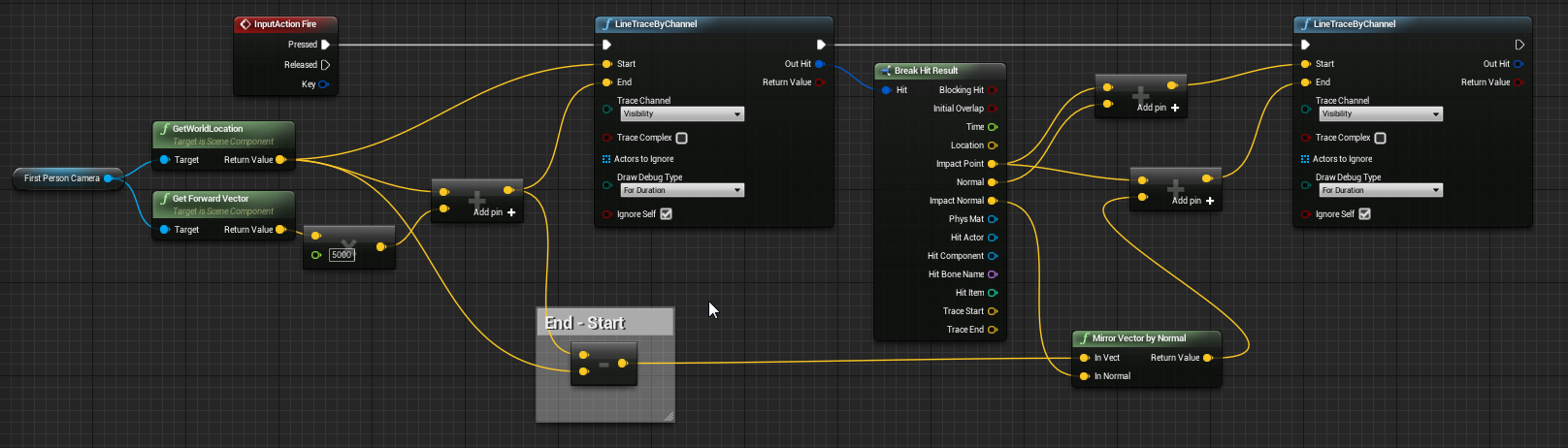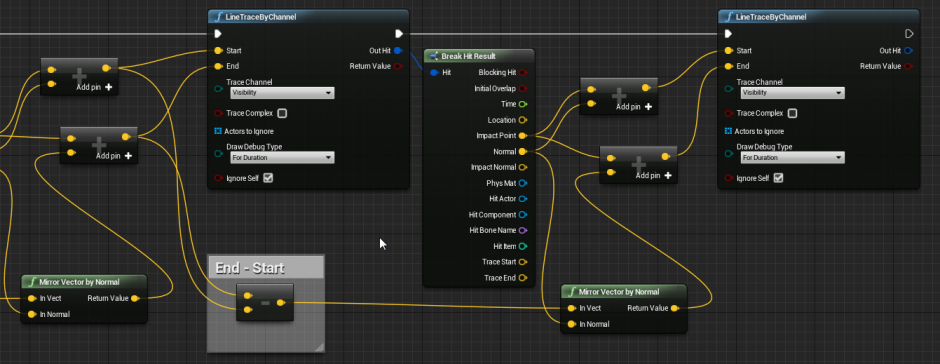# Legacy/Simple Line Trace Ricochet

Overview This tutorial will show you how to create a blueprint that will allow the player to shoot a line trace (ray trace) that will ricochet off of surfaces. More line traces can be strung togeth...

## Overview

This tutorial will show you how to create a blueprint that will allow the player to shoot a line trace (ray trace) that will ricochet off of surfaces. More line traces can be strung together to ricochet as many times as needed.

To do this you will need to follow this logic:

1. On key press, trace a line from the player until an object is hit

2. If an object is hit, get the Impact Point and Impact Normal

3. Mirror the vector of the original line trace

4. Then create another line trace from the Normal and Impact PointFile:Ricochet BP.png

## Setting up the blueprint

1. Open the Character blueprint and go to the Event Graph

`    a. Right-click and create a ``Line`` ``Trace`` ``by`` ``Channel`` node & set the ``Draw`` ``Debug`` ``Type`` to ``For`` ``Duration``.`

2. Getting the player info

`    a. In the bottom of the ``MyBlueprint`` panel, check ``Show`` ``Inherited`` ``Variables``.`
`    b. Find the ``FirstPersonCamera`` and drag a ``Get`` variable into the graph.`
`    c. Drag off of the camera variable and find the ``Get`` ``World`` ``Location`` node (``Context`` ``Sensitivity`` may need to be off).`
`    d. Plug the return value into the ``Start`` pin of the line trace.`
`    e. Drag off of the camera variable and find the ``Get`` ``Forward`` ``Vector`` node.`
`    f. Multiply the forward vector by 5000 (or any other value you like, this is the distance the line trace will go).`
`    g. Add the vectors from the world location and the multiplied value.  Plug the result into the ``End`` pin of the line trace.`
`    h. Subtract the ``Return`` ``Value`` from the ``Get`` ``World`` ``Location`` node from the value from the previous added node (End - Start in the picture above.)`

3. Getting the hit infomation and the second line trace

`    a. Drag off of the ``Out`` ``Hit`` pin of the line trace & create a ``Break`` ``Hit`` ``Result`` node.`
`    b. Create another ``Line`` ``Trace`` ``by`` ``Channel`` node and set it ``For`` ``Duration``.`
`    c. Drag off of the ``Impact`` ``Point`` pin of the break hit and create an ``Add`` node for Vector + Vector.`
`       (Note: Attempting to reflect 'exactly' off of a normal will sometimes have errors. This adds 1, depending on normal direction, to the next line trace to make sure it is not inside of the object.)`
`    d. Connect the ``Normal`` to the ``Add`` node and connect the output to the Start pin of the next line trace.`
`    e. Drag off of the result from the End - Start calculation from earlier & get a ``Mirror`` ``Vector`` ``by`` ``Normal`` node.`
`    f. Connect the ``Impact`` ``Normal`` pin of the break hit to the ``In`` ``Normal`` pin of the mirror vector.`
`    g. Drag off of the ``Impact`` ``Point`` pin of the break hit and create another ``Add`` node for Vector + Vector`
`    h. Connect the ``Return`` ``Value`` pin of the ``Mirror`` ``Vector`` ``by`` ``Normal`` node to the ``Add`` node that was just created and plug the result into the ``End`` pin`

4. Stringing more line traces together

`    a. Select the last 3 nodes (``Break`` ``Hit`` ``Result``, ``Mirror`` ``Vector`` ``by`` ``Normal``, and ``Line`` ``Trace`` ``by`` ``Channel``).`
`    b. Copy them to the end of the blueprint.`File:Ricochet BP2.png

`    c. Connect the ``Execute`` pins for the line traces.`
`    d. Connect the ``Out`` ``Hit`` pin to the new ``Break`` ``Hit`` ``Result``.`
`    e. Create another End - Start calculation with the values from the previous line trace and connect the output to the new ``Mirror`` ``Vector`` ``by`` ``Normal`` node.`
`    f. Follow the steps above to set up the same line trace again as many time as needed`

NOTE: This tutorial has been tested and works correctly with version 4.10.4 of the editor.

Cheers,

TJ Ballard & Matthew Clark